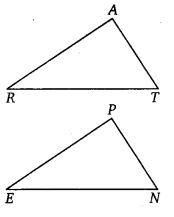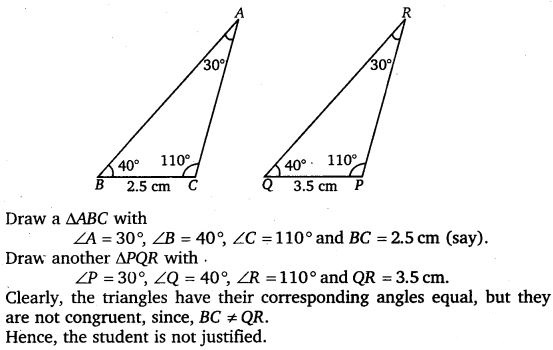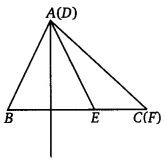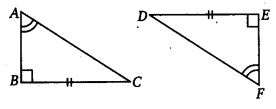# Class 7 Maths NCERT Solutions for Chapter – 7 Congruence of Triangles Ex – 7.2

## Congruence of Triangles

Question 1.
Which congruence criterion do you use in the following ?Solution:

Question 2.
You want to show that ∆ART = ∆PEN.(a) If you have to use SSS criterion, then you need to

1. AR =
2. RT =
3. AT =

(b) If it is given that ∠T = ∠N and you are to use SAS criterion, you need to have

1. RT = and,
2. PN =

(c) If it is given that AT = PN and you are to use ASA criterion, you need to have

1. ?
2. ?

Solution:
(a) In order to show that ∆ART = ∆PEN using SSS criterion, we need to show that

1. AR = PE
2. RT = EN
3. AT = PN

(b) If m∠T = m∠N and to use SAS criterion, we need to show that

1. RT = EN and
2. PN = AT

(c) If AT = PN and to use ASA criterion, we need to show that

1. ∠RAT = ∠EPN and
2. ∠ATR = ∠PNE

Question 3.

Solution:

Question 4.
In ∆ABC, ∠A = 30°, ∠B = 40° and ∠C = 110°
In ∆PQR, ∠P = 30°, ∠Q = 40° and ∠R = 110°.
A student says that ∆ABC = ∆PQR by AAA congruence criterion. Is he justified? Why or why not?

Solution:
In two triangles, if the three angles of one triangle are respectively equal to the three angles of the other, then the triangles are not necessarily congruent.Question 5.
In the figure, the two triangles are congruent The corresponding parts are ∆ RAT = ?Solution:

Question 6.
Complete the congruence statement :Solution:

Question 7.
In a squared sheet, draw two triangles of equal areas such that
(i)
the triangles are congruent.
(ii) the triangles are not congruent.
What can you say about their perimeters?
Solution:

Question 8.
Draw a rough sketch of two triangles such that they have five pairs of congruent parts but still the triangles are not congruent.

Solution:
In some special cases (which depend on the lengths of the sides and the size of the angle involved),

SSA is enough to show congruence. However, it is not always enough. Consider the following triangles :Here side AB is congruent to side DE (S) side AC is congruent to side DF(S) angle C is congruent to angle F(A)

But the triangles are not congruent, as we can see.

What happens is this : If we draw a vertical line through point A in the first triangle, we can sort of “flip” side AB around this line to get the second triangle. If we were to lay one triangle on top of the other and draw the vertical line, this how it would look.Clearly, side DE is just side AB flipped around the line. So, we have not changed the length of the side, and the other side AC (or DF) is unchanged, as is angle C (or F). So, these two triangles that have the same SSA information, but they are not congruent.

Question 9.
If ∆ABC and ∆PQR are to be congruent, name one additional pair of corresponding parts. What criterion did you use?Solution:
In order prove that ∆ABC ≅ ∆PQR, the additional pair of corresponding parts are named as BC = QR.
Criterion used is ASA rule of congruence

Question 10.
Explain, why ∆ABC ≅ ∆FEDSolution: